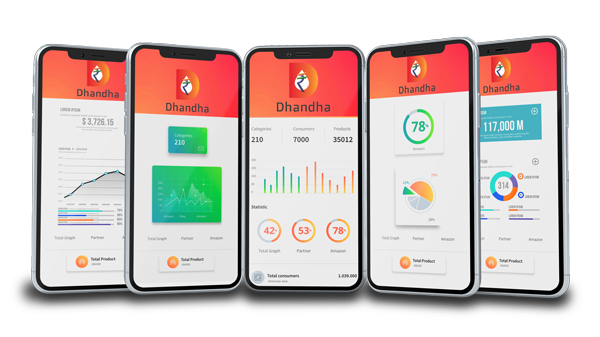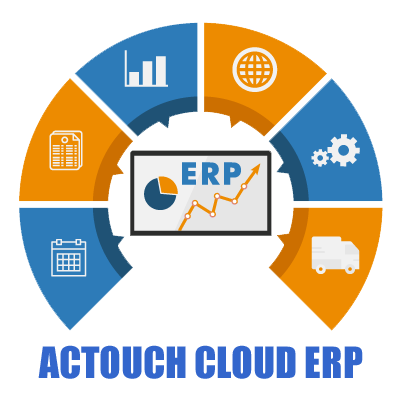# Discussion Community

Cloud Web-based ERP SaaS Software & GST

# What is Depreciation? Different types of Depreciation Methods.

(@dheeraj)
Member
Joined: 3 years  ago
Posts: 65
November 28, 2017 4:49 pm

What is Depreciation? Different types of Depreciation Methods.

Depreciation is a decrease in the value of a fixed (capital) asset (a piece of equipment, a building, a vehicle, etc.) over the time that the asset is being used.

• Events that can cause assets to depreciate include wear and tear, usage, age, deterioration, obsolescence (change in technology) and accidents.

Measuring the loss in value of an asset is known as depreciation.

• The International Accounting Committee defines depreciation as follows: Depreciation is the allocation of the depreciable amount of an asset over its estimated useful life. Useful life is the period over which a depreciable asset is expected to be used by the enterprise.

What Can Depreciate?

• Vehicles
• Office furniture
• Office equipment
• Buildings you own
• Machinery you use to manufacture products

What Can’t Depreciate:

• Land, Inventory

Different types of Depreciation Methods.

Four basic methods exist for computing depreciation:

1) Straight-line Depreciation

2) Units of production Depreciation

3) Double Declining balance Depreciation

4) Sum-of-the-years digits Depreciation

• Straight-line Depreciation

Straight Line - Depreciation Formula Example

Pensive Corporation purchases the Procrastinator Deluxe machine for \$60,000. It has an estimated salvage value of \$10,000 and a useful life of five years. Pensive calculates the annual straight-line depreciation for the machine as:

1. Purchase cost of \$60,000 – estimated salvage value of \$10,000 = Depreciable asset cost of \$50,000
2. 1 / 5-year useful life = 20% depreciation rate per year
3. 20% depreciation rate x \$50,000 depreciable asset cost = \$10,000 annual depreciation

• Units of production - Depreciation Formula Example

The following formula is used to calculate depreciation under this method:

 Depreciation = Number of Units Produced × (Cost − Salvage Value) Life in Number of Units

Depreciation = (15/150) × (\$110 million - \$10 million) = \$10 million

• Double Declining balance - Depreciation Formula Example

Example of Double Declining Balance Depreciation

ABC Company purchases a machine for \$100,000. It has an estimated salvage value of \$10,000 and a useful life of five years. The double declining balance depreciation calculation is:

 Year Net book value, beginning of year Double-declining balance depreciation computed as 2 × SL  rate × beginning NBV Net book value, end of year 1 \$100,000 \$40,000 \$60,000 2 60,000 24,000 36,000 3 36,000 14,400 21,600 4 21,600 8,640 12,960 5 12,960 2,960 10,000 salvage value Total \$90,000

• Sum-of-the-years digits Depreciation Formula Example.

Example of Sum of the Years' Digits Depreciation

ABC Company purchases a machine for \$100,000. It has an estimated salvage value of \$10,000 and a useful life of five years. The sum of the years' digits depreciation calculation is:

 SYD = 5(5 + 1) = 15 2

This formula yields the sum of each year of the estimated useful life:

1 + 2 + 3 + 4 + 5 = 15

 Year Remaining estimated useful  life at beginning of year SYD Applicable percentage Annual depreciation 1 5 5/15 33.33% \$30,000 2 4 4/15 26.67 24,000 3 3 3/15 20.00 18,000 4 2 2/15 13.33 12,000 5 1 1/15 6.67 6,000 Totals 15 100.00% \$90,000

Share:
CLOUD ERP
ACCOUNTING
HANDLES ALL TYPES OF ACCOUNTING TRANSACTIONS
INVENTORY
TRACK FLOW OF GOODS FROM MANUFACTURERS TO WAREHOUSES
BENEFITS
INCREASE BUSINESS INSIGHTS AND STREAMLINE OPERATIONS, COST EFFECTIVE, FAST IMPLEMENTATIONDHANDHA INVOICE AND BILLING APP

* Create Invoice in 2 min and send the materials
* Enter your Bills on move
* Track your Payments and Receipts
* GST Returns
* Reports
* Bank Level Data Security
* Tally Software Interface
AUTOMATE YOUR BUSINESS PROCESS WITH ACTOUCH CLOUD ERP AT AN UNBELIEVABLE PRICE
• Improved Process Efficiency
• Accurate Forecasting
• Department Collaboration
• Scalable Resource
• Integrated Information
• Cost Savings
• Streamlined Processes
• Mobility
• Customized Reporting
• Increased Productivity
• Regulatory Compliance
• Flexible Systems
• Customer Service
• Data ReliabilityWe hate spam and never share your details.AUTOMATE YOUR BUSINESS PROCESS WITH ACTOUCH CLOUD ERP AT AN UNBELIEVABLE PRICE
• Improved Process Efficiency
• Accurate Forecasting
• Department Collaboration
• Scalable Resource
• Integrated Information
• Cost Savings
• Streamlined Processes
• Mobility
• Customized Reporting
• Increased Productivity
• Regulatory Compliance
• Flexible Systems
• Customer Service
• Data Reliability

Working